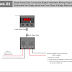Hey, in this article we are going to see both single-phase and three-phase voltmeter wiring diagrams and connection procedures. Also, we will see the wiring diagram for using a single-phase digital voltmeter to measure the three-phase voltage by connecting a Selector Switch. These Voltmeters are used in electrical panels so they are also known as panel voltmeters. Basically, A Voltmeter is a measuring device that helps to measure the electrical potential or voltage. In older days, analog voltmeters are used, where a pointer moves to show the measurement value. Digital voltmeters are advanced devices, they show the measured value in digital form, so any user can see the exact measurement value in any fraction.

## Single Phase Digital Voltmeter Wiring Diagram

Here, you can see the wiring diagram of a single-phase(two-terminal) digital voltmeter.

Here, the above voltmeter has a voltage input range from 50V to 480V. So, we can measure any voltage between this range. We know that our single-phase voltage(230V) and phase-to-phase voltage(440V) of a three-phase comes under this range, so we can measure these voltages also. Generally, single-phase voltmeters are mostly used for single-phase voltages(Ex: 230V).

Connection Procedure
1. Here, the voltmeter is designed for a 230V AC auxiliary supply. So, connect a 230V AC power source to its power supply terminals. Remember that this auxiliary always be turned on during the measurement.
2. Now connect the voltage source to be measured to the voltmeter.
3. If you want to measure a single-phase voltage then connect the phase and neutral terminal of the voltage source to the voltmeter.
4. If you want to measure line-to-line voltage of any three-phase system then connect the two line terminals to the voltmeter. Must check your voltmeter range if it is able to measure up to 480V.

## Single Phase Digital Voltmeter Connection with Selector Switch

Here, you can see the connection diagram between Single Phase Digital Voltmeter and Selector Switch for Three Phase Voltage Measurement.

Here, you can measure all the three-phase voltages such as Line to Line voltage and Line to neutral voltages by rotating the selector switch. Must ensure that your voltmeter is designed to measure voltage up to 480V. Otherwise, your voltmeter will be burned.

Connection Procedure
1. Connect the auxiliary power supply to the voltmeter.
2. Connect the output terminals(V1 and V2) of the selector switch to the voltage input terminals of the voltmeter. The V1 terminal should be connected to the phase terminal and the V2 terminal should be connected to the Neutral Terminal.
3. Now, connect the three-phase voltage source to be measured to the selector switch. Connect R, Y, and B terminals to the L1, L2, and L3 terminals respectively, and connect the neutral terminal to the 'N' terminal. If neutral is not available then kept it disconnected.
4. After completing all the connections turn on the auxiliary power supply and the three-phase voltage source to be measured.

## Three-Phase Digital Voltmeter Wiring Diagram

Here, you can see the Wiring Diagram of Three Phase Digital Voltmeter.

Here, the voltmeter will show all the Line to Line and Line to Neutral Voltages on the display with a time delay. Or you can check manually all the voltages by pressing the switch on it.

Connection Procedure
1. Connect the auxiliary power supply to the voltmeter.
2. Connect all the phases and neutral terminals of the three-phase voltage source to be measured to the voltage input terminals of the voltmeter.
3. Remember that all the connections should be done as per the identification of the voltmeter. Don't interchange any phases otherwise, the voltmeter will show the wrong values. Also, don't connect any line terminals to the neutral terminal of the voltmeter. The voltmeter will damage because the neutral is able to take low voltage input only.

Digital Voltmeter Wiring Diagram and Connection ProcedureReviewed by Author on June 08, 2023 Rating: 5